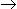# Aptitude - Numbers

Exercise : Numbers - General Questions
6.
How many of the following numbers are divisible by 132 ?
264, 396, 462, 792, 968, 2178, 5184, 6336
4
5
6
7
Explanation:

132 = 4 x 3 x 11

So, if the number divisible by all the three number 4, 3 and 11, then the number is divisible by 132 also.

26411,3,4 (/)

39611,3,4 (/)

46211,3 (X)

79211,3,4 (/)

96811,4 (X)

217811,3 (X)

51843,4 (X)

633611,3,4 (/)

Therefore the following numbers are divisible by 132 : 264, 396, 792 and 6336.

Required number of number = 4.

7.
(935421 x 625) = ?
575648125
584638125
584649125
585628125
Explanation:

 935421 x 625 = 935421 x 54 = 935421 x104 2

 = 935421 x 104 = 9354210000 24 16

= 584638125

8.
The largest 4 digit number exactly divisible by 88 is:
9944
9768
9988
8888
None of these
Explanation:
```Largest 4-digit number = 9999

88) 9999 (113
88
----
119
88
----
319
264
---
55
---

Required number = (9999 - 55)
= 9944.
```

9.
Which of the following is a prime number ?
33
81
93
97
Explanation:
Clearly, 97 is a prime number.

10.
What is the unit digit in {(6374)1793 x (625)317 x (341491)}?
2
3
5